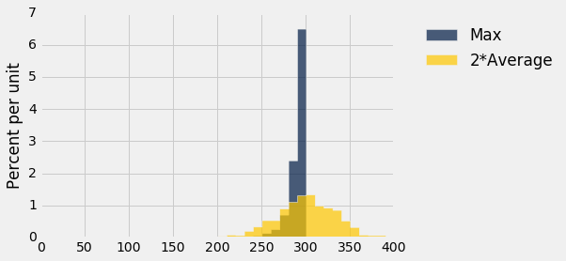# 九、经验分布

``````die = Table().with_column('Face', np.arange(1, 7, 1))
die
``````
Face
1
2
3
4
5
6

### 概率分布

``````die_bins = np.arange(0.5, 6.6, 1)
die.hist(bins = die_bins)
``````### 经验分布

``````die.sample(10)
``````
Face
5
3
3
4
2
2
4
1
6
6

``````def empirical_hist_die(n):
die.sample(n).hist(bins = die_bins)
``````

### 经验直方图

``````empirical_hist_die(10)
``````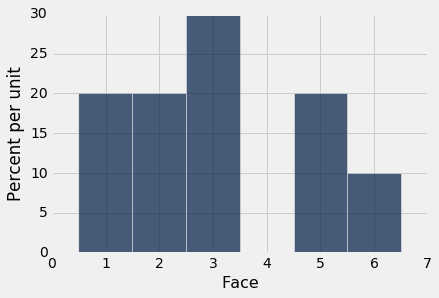``````empirical_hist_die(100)
``````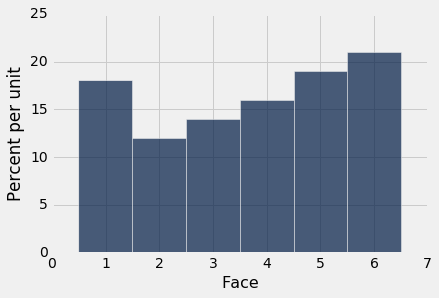``````empirical_hist_die(1000)
``````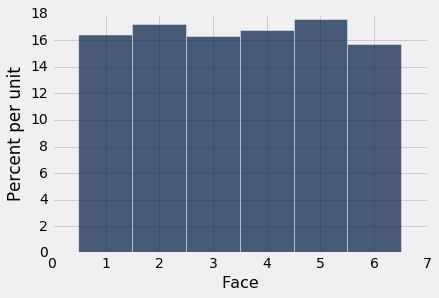## 从总体中取样

``````united = Table.read_table('united_summer2015.csv')
united
``````
Date Flight Number Destination Delay
6/1/15 73 HNL 257
6/1/15 217 EWR 28
6/1/15 237 STL -3
6/1/15 250 SAN 0
6/1/15 267 PHL 64
6/1/15 273 SEA -6
6/1/15 278 SEA -8
6/1/15 292 EWR 12
6/1/15 300 HNL 20
6/1/15 317 IND -10

（省略了 13815 行）

``````united.column('Delay').min()
-16

united.column('Delay').max()
580

delay_bins = np.append(np.arange(-20, 301, 10), 600)
united.select('Delay').hist(bins = delay_bins, unit = 'minute')
``````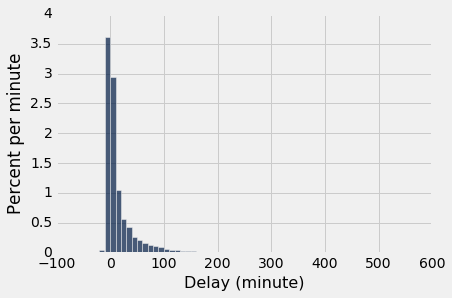``````united.where('Delay', are.above(200)).num_rows/united.num_rows
0.008390596745027125

delay_bins = np.arange(-20, 201, 10)
united.select('Delay').hist(bins = delay_bins, unit = 'minute')
``````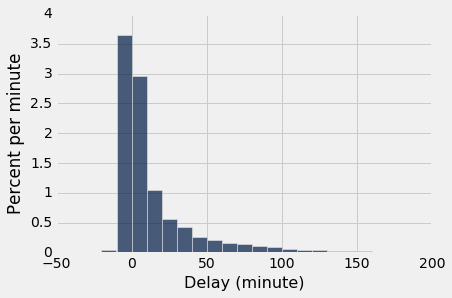`[0,10)`的条形高度不到每分钟 3%，这意味着只有不到 30% 的航班延误了 0 到 10 分钟。 这是通过行的计数来确认的：

``````united.where('Delay', are.between(0, 10)).num_rows/united.num_rows
0.2935985533453888
``````

### 样本的经验分布

``````def empirical_hist_delay(n):
united.sample(n).select('Delay').hist(bins = delay_bins, unit = 'minute')
``````

``````empirical_hist_delay(10)
``````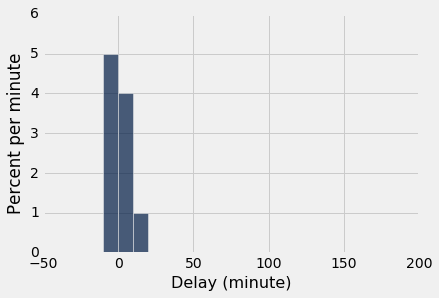``````empirical_hist_delay(100)
``````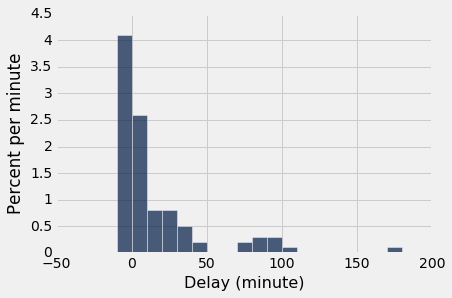``````empirical_hist_delay(1000)
``````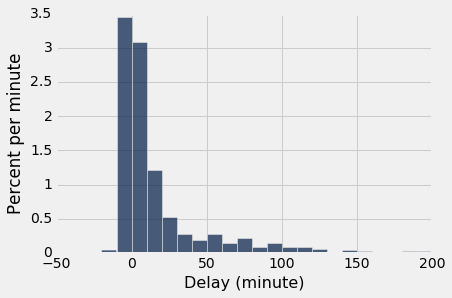## 轮盘赌

`wheel`表代表内华达轮盘赌的口袋。

``````wheel
``````
Pocket Color
0 green
00 green
1 red
2 black
3 red
4 black
5 red
6 black
7 red
8 black

（省略了 28 行）

``````def red_winnings(color):
if color == 'red':
return 1
else:
return -1
bets = wheel.with_column(
'Winnings: Red', wheel.apply(red_winnings, 'Color')
)
bets
``````
Pocket Color Winnings: Red
0 green -1
00 green -1
1 red 1
2 black -1
3 red 1
4 black -1
5 red 1
6 black -1
7 red 1
8 black -1

（省略了 28 行）

``````one_spin = bets.sample(1)
one_spin
``````
Pocket Color Winnings: Red
14 red 1

``````num_simulations = 5000

colors = make_array()
winnings_on_red = make_array()

for i in np.arange(num_simulations):
spin = bets.sample(1)
new_color = spin.column("Color").item(0)
colors = np.append(colors, new_color)
new_winnings = spin.column('Winnings: Red')
winnings_on_red = np.append(winnings_on_red, new_winnings)

Table().with_column('Color', colors)\
.group('Color')\
.barh('Color')
``````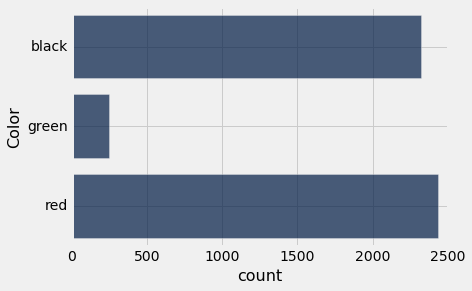38 个口袋里有 18 个是红色的，每个口袋都是等可能的。 因此，在 5000 次模拟中，我们预计大致（但可能不是完全）看到`18/38*5000`或者 2,368 次红色。模拟证明了这一点。

``````Table().with_column('Winnings: Red', winnings_on_red)\
.hist(bins = np.arange(-1.55, 1.65, .1))
``````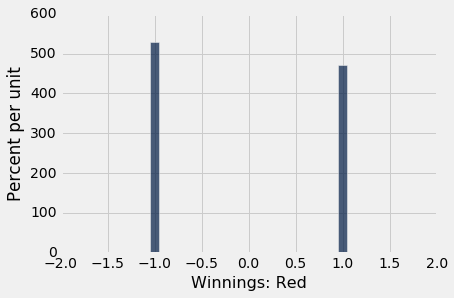### 多次游戏

``````spins = bets.sample(200)
spins.column('Winnings: Red').sum()
-26
``````

``````num_spins = 200

net_gain = make_array()

for i in np.arange(num_simulations):
spins = bets.sample(num_spins)
new_net_gain = spins.column('Winnings: Red').sum()
net_gain = np.append(net_gain, new_net_gain)

Table().with_column('Net Gain on Red', net_gain).hist()
```````split_winnings`函数将口袋作为参数，如果口袋是 0 或 00，则返回 17。对于所有其他口袋，返回 -1。

``````def split_winnings(pocket):
if pocket == '0':
return 17
elif pocket == '00':
return 17
else:
return -1
more_bets = wheel.with_columns(
'Winnings: Red', wheel.apply(red_winnings, 'Color'),
'Winnings: Split', wheel.apply(split_winnings, 'Pocket')
)
more_bets
``````
Pocket Color Winnings: Red Winnings: Split
0 green -1 17
00 green -1 17
1 red 1 -1
2 black -1 -1
3 red 1 -1
4 black -1 -1
5 red 1 -1
6 black -1 -1
7 red 1 -1
8 black -1 -1

（省略了 28 行）

``````net_gain_red = make_array()
net_gain_split = make_array()

for i in np.arange(num_simulations):
spins = more_bets.sample(num_spins)
new_net_gain_red = spins.column('Winnings: Red').sum()
net_gain_red = np.append(net_gain_red, new_net_gain_red)
new_net_gain_split = spins.column('Winnings: Split').sum()
net_gain_split = np.append(net_gain_split, new_net_gain_split)

Table().with_columns(
'Net Gain on Red', net_gain_red,
'Net Gain on Split', net_gain_split
).hist(bins=np.arange(-200, 200, 20))
``````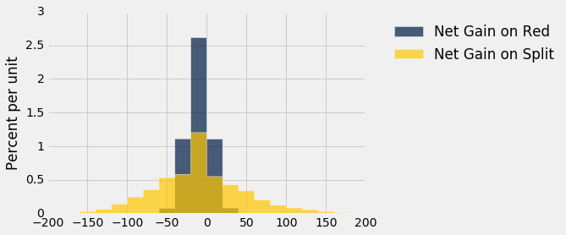## 统计量的经验分布

``````united = Table.read_table('united_summer2015.csv')
delay_bins = np.arange(-20, 201, 10)
united.select('Delay').hist(bins = delay_bins, unit = 'minute')
plots.title('Population');
``````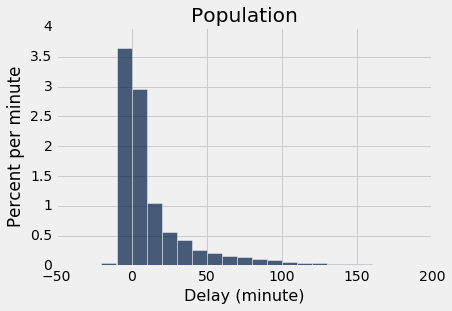``````sample_1000 = united.sample(1000)
sample_1000.select('Delay').hist(bins = delay_bins, unit = 'minute')
plots.title('Sample of Size 1000');
``````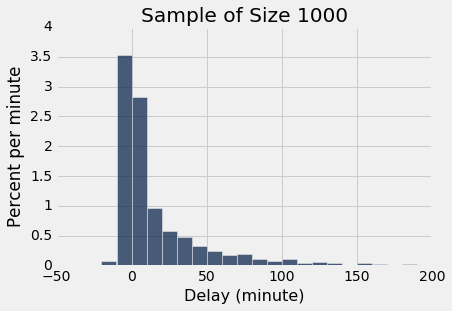### 参数

``````np.median(united.column('Delay'))
2.0
``````

NumPy 函数`median`返回数组的中值（中位数）。 在所有的航班中，延误时间的中位数为 2 分钟。 也就是说，总体中约有 50% 的航班延误了 2 分钟以内：

``````united.where('Delay', are.below_or_equal_to(2)).num_rows/united.num_rows
0.5018444846292948
``````

``````united.where('Delay', are.equal_to(2)).num_rows
480
``````

### 统计

``````np.median(sample_1000.column('Delay'))
2.0
``````

``````np.median(united.sample(1000).column('Delay'))
3.0
``````

### 模拟统计量

• 上面的第一步是`for`循环的主体。
• 第二步，重复第一步“无数次”，由循环完成。 我们“无数次”是5000次，但是你可以改变这个。
• 第三步是显示表格，并在后面的单元格中调用`hist`

``````medians = make_array()

for i in np.arange(5000):
new_median = np.median(united.sample(1000).column('Delay'))
medians = np.append(medians, new_median)

Table().with_column('Sample Median', medians)
``````
Sample Median
3
2
2
3
2
2
2
3
1
3

（省略了 4990 行）

``````Table().with_column('Sample Median', medians).hist(bins=np.arange(0.5, 5, 1))
``````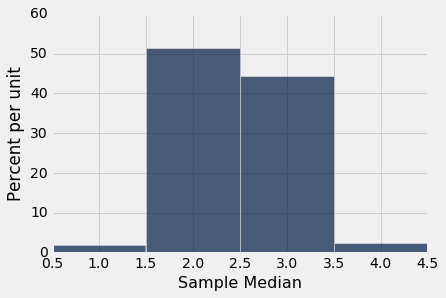### 估计敌军飞机的数量

• 战机有`N`架，编号为 `1,2, ..., N`

• 观察到的飞机从`N`架飞机中均匀、随机带放回地抽取。

``````N = 300
serialno = Table().with_column('serial Number', np.arange(1, N+1))
serialno
``````
serial number
1
2
3
4
5
6
7
8
9
10

（省略了 290 行）

``````serialno.sample(30).column(0).max()
291
``````

### 模拟统计

``````sample_size = 30
repetitions = 750
maxes = make_array()

for i in np.arange(repetitions):
sampled_numbers = serialno.sample(sample_size)
maxes = np.append(maxes, sampled_numbers.column(0).max())

Table().with_column('Max Serial Number', maxes)
``````
Max Serial Number
280
253
294
299
298
237
296
297
293
295

（省略了 740 行）

``````every_ten = np.arange(1, N+100, 10)
Table().with_column('Max Serial Number', maxes).hist(bins = every_ten)
``````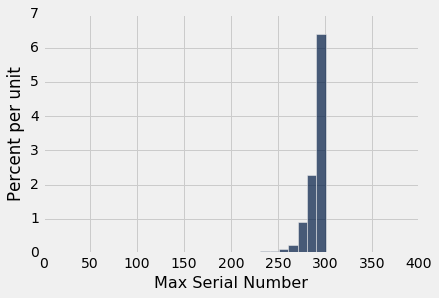### 良好的近似

``````300**30
205891132094649000000000000000000000000000000000000000000000000000000000000
``````

### 参数的不同估计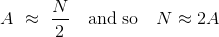``````maxes = make_array()
twice_ave = make_array()

for i in np.arange(repetitions):
sampled_numbers = serialno.sample(sample_size)

new_max = sampled_numbers.column(0).max()
maxes = np.append(maxes, new_max)

new_twice_ave = 2*np.mean(sampled_numbers.column(0))
twice_ave = np.append(twice_ave, new_twice_ave)

results = Table().with_columns(
'Repetition', np.arange(1, repetitions+1),
'Max', maxes,
'2*Average', twice_ave
)

results
``````
Repetition Max 2*Average
1 296 312.067
2 283 290.133
3 290 250.667
4 296 306.8
5 298 335.533
6 281 240
7 300 317.267
8 295 322.067
9 296 317.6
10 299 308.733

（省略了 740 行）

``````results.drop(0).hist(bins = every_ten)
``````